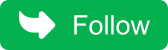## Wednesday, May 4, 2022

### Question 49: How to find middle element of linked list.

One of the algo for this would be:

• Traverse the list and find the length of list
• After finding length, again traverse the list and locate n/2 element from head of linkedlist.

Time complexity=time for finding length of list + time for locating middle element=o(n)+o(n) =o(n)
Space complexity= o(1).

### Use two pointer fastptr and slowptr and initialize both to head of linkedlist

1. Move fastptr by two nodes and slowptr by one node in each iteration.
2. When fastptr reaches end of nodes, the slowptr pointer will be  pointing to middle element.

Lets create java program for it:

``````public class LinkedList{

private static class Node {
private int value;
private Node next;

Node(int value) {
this.value = value;

}
}

} else {
while (temp.next != null)
temp = temp.next;

temp.next = node;
}
}

public void printList() {
while (temp != null) {
System.out.format("%d ", temp.value);
temp = temp.next;
}
System.out.println();
}

// This function will find middle element in linkedlist
{
Node slowPointer, fastPointer;

while(fastPointer !=null) {
fastPointer = fastPointer.next;
if(fastPointer != null && fastPointer.next != null) {
slowPointer = slowPointer.next;
fastPointer = fastPointer.next;
}
}

return slowPointer;

}

public static void main(String[] args) {

list.printList();
// finding middle element
System.out.println("Middle node will be: "+ middle.value);

}

}
``````

Logically linked list can be represented as:

The middle element is represented in red color.
Run the above program, and you will get the following output:

``````5 6 7 1 2
Middle node is: 7``````
Don't miss the next article!

Be the first to be notified when a new article or Kubernetes experiment is   published.